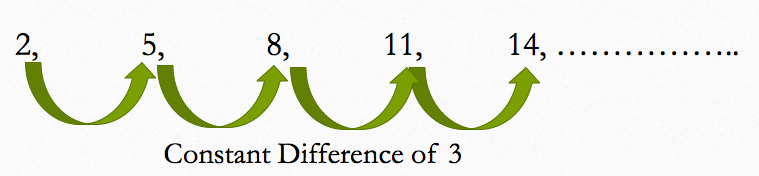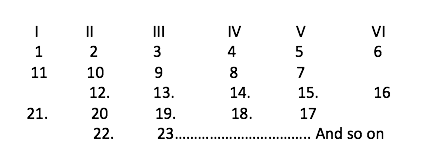# Algebra

#### FUNCTIONS-1

Sequence and Series - 1

Sequence and Series:

In mathematics, sequence is a list of numbers or objects arranged in a particular manner or in a sequential order while series means the sum of the sequence. In this chapter, one by one we will study different types of series which usually appear in competitive exams.

1. Arithmetic Progression:

In an arithmetic progression or popularly known as A.P., the difference of consecutive terms is constant. For e.g. consider the series given below:

2, 5, 8, 11, 14, …….Here the difference between consecutive terms is 3 which is constant.

So, general form of an A.P. is  a, a+d , a+2d, a+3d, …….. a+(n-1)d

Where a is the first term and d is the common term.

So, nth term of an A.P. is given by the formula:Note: if there are three numbers, a,b and c in A.P., then

b – a = c- b  or 2b = a+c

If three numbers are in A.P., then a better approach is to assume the numbers to be a-d, a and a+d

Similarly if four numbers are in A.P., then assume these numbers to a-3d, a-d, a+d and a+3d.

Sum of n terms of an A.P.

Let us say that a given A.P. is of the form :  a, a+d, a+2d, a+3d,……. a+(n-1)d ;

Then the sum of the 1st term and last term = a + a +(n-1)d = 2a +(n-1)d

Sum of the 2nd term and the second last = a+d + a +(n-2)d = 2a + (n-1)d

And so on

Thus, the sum of equidistant terms is constant in an A.P.

So, sum of all the terms = 1/2 * (number of terms ) (First term + Last term)

= (n/2) *{2a + (n-1)d}Example 1: Three numbers are in A.P. and their sum is 36. If the sum of their squares is 482, then what are the three numbers?

Solution: Let the three numbers be a-d, a and a+d.

So, a-d +a +a+d = 36   or a = 12

So, the numbers are 12-d, 12 and 12+d

Also, (12-d)2 + 122 + (12+d)2 = 482

144 +d2 -24d + 144 + 144 + d2 +24d = 482

2d2 = 50 or d = ±5

Thus, the given numbers are 7,12 and 17.

Example 2: Divide 168 into four parts such that they are in A.P. and twice the product of the smallest part and the largest part is 64 more than the product of the second smallest and third smallest part.

Solution: Let the four numbers be a-3d, a-d, a+d and a+3d

Sum of these numbers is: 4a

So, 4a = 168 or a = 42

Also, 2(a-3d)(a+3d) = 64 + (a-d)(a+d)

2( a2 – 9d2) = 64 + a2 –d2

422 -64 = 17d2

or d = 10

Thus, the four numbers are 12, 32, 52 and 72

Example 3.Find the sum of all natural numbers between 100 and 300 which leave a remainder of 3 when divided by 5 in each case.

Solution: Any number which gives a remainder of 3 when divided by 5 will be of the form 5n+3,

Here the numbers must lie between 100 and 300

So, the smallest such number is 103 and the largest such number will be 298.All the remaining numbers will be present at a common difference of 5.

To find the sum we’ll first calculate the number of terms

298 = 103 + (n-1)*5

or n = 40

Sum = 402*(103 +298)    = 8020

Example 4. An A.P. consists of even number of terms. The sum of all the odd numbered terms is 36 while that of all the even numbered terms is 48. If the last term exceeds the first term by 23.25, then how many terms are there in the series?

Solution: Let the sequence has 2n terms.

a1 + a3 + a5 +…….. a2n-1 = 36  (1)

a2 + a4 + a6 + ……..a2n = 48    (2)

Subtract (1) from (2), we get

d + d+…….+d (upto n terms ) = 12

or nd = 12    (3)

Also, a2n – a1 = (2n-1)d

23.25 = (2n-1)d     (4)

From (3) and (4) we get n = 16

Thus, there are 16 terms in the given sequence.

Example 5: Let the sum of p,2p and 3p terms of an A.P. be A, B and C respectively. Show that C = 3(B-A)Example 6: The number of common terms in the two sequences 17, 21, 25, ….. 417 and 16, 21, 26, ….., 466 is     (CAT 2008)

1. 78         2. 19         3. 20         4. 77         5. 22

Solution : Let S1: 17,21,25,……..417  and

S2 : 16,21,26,…….466

The common difference of S1 is 4 while that of S2 is 5 and the first common term in both the series is 21

So, the series with common terms will also be an A.P with a common difference of L.C.M. of (4 &5) = 20

So, terms will be 21, 41,61,…… i.e. to say the terms are of the form 20k+1. Thus, the last common term will be 401.

Let the number of common terms be n

So, 401 = 21 + (n-1)20

Or n = 20

Thus, option (3) is correct.

Example 7: The sum of 3rd and 15th elements of an arithmetic progression is equal to the sum of 6th, 11th and 13th elements of the same progression. Then which element of the series should necessarily be equal to zero? (CAT 2003)

1. 1st         2. 9th         3. 12th             4. None of the above.

Solution: Let the first term of the A.P.be a and the common difference be d

So, a+2d + a +14d = a+5d +a+10d +a+12d

Or a = -11d

Thus a+11d = 0 which is the 12th term in the given sequence.

Hence, option (3) is correct.

Example 8: If log32, log3 (2x – 5), log3 (2x – 7/2) are in arithmetic progression, then the value of x is equal to (CAT 2003):

1. 5         2. 4         3. 2         4. 3

Solution: Given that: log32, log3 (2x – 5), log3 (2x – 7/2) are in A.P.

So, 2log3(2x -5) = log32 + log3(2x -7/2)

Or log3(2x-5)2 = log3{2*(2x -7/2)}

Or (2x -5)2 = 2(2x -7/2)

Solving the equation, we get x = 2 or 3

But x cannot be 2 as that will make 2x -5 negative. So, x = 3

Hence, option (4) is correct.

Example 9: The numbers are written in the following manner :In which column does the number 2014 lie? Also in which particular row does it lie?

Solution: The numbers in the column I are 1,11,21,…. i.e. of the form 10k+1

Similarly the numbers in column VI are 6,16, 26,…..i.e. of the form 10k+6.

The numbers in column II are 2,12,22- of form 10k+2 and 10,20,.. of the form 10k

The numbers in column III are 3,13,23 – of the form 10k+3 and 9,19,.. of the form 10k+9

The numbers in column IV are of the from of either 10k+4 or 10k+8 and similarly the numbers in column V are of the form 10k+5 or 10k+7.

Now, 2014 is of the form 10k+4, so it lies in column IV.

Now, consider the series 4,14,24,… 2014

2014 = 4 + (n-1)10

or n= 202

But it doesn’t lie in 202th row. For eg, 14 is the second term but lies in the 3rd row, similarly 24 is the 3rd term of the series but it lies in the fifth row. So, row number becomes (2n-1) where n is the position of the term in the series (4,14,24,….)

So, required row number = (2*202-1) = 403

Hence, 2014 lies in the IV column and 403rd row.

Arithmetic Mean (A.M):

If there are two numbers a and b and if we need to find the arithmetic mean of these two numbers, then A.M.  would be equal to (a+b)/2

In general if we want to calculate the arithmetic mean of n numbers- a1,a2,…an,

Then, Arithmetic Mean = a1+a2+a3+……..ann

Inserting Arithmetic Means between two Numbers:

Let us say we want to insert n arithmetic means between two numbers a and b

Let these arithmetic means be a1,a2,a3,…..an

Now, this means that a,a1,a2,……an, b are all in A.P, with the first term as a, the last term as b and the number of terms as n +2 { including a and b}

b = a + (n+2-1)d  or d = (b-a)/(n+1)

So, a1 = a +d = a + (b-a)/(n+1)

a2 = a + 2d = a+ 2(b-a)/(n+1)

And so on we can calculate the n arithmetic means till an.

Example 10: Between two numbers 3 and 11, 15 arithmetic means are inserted. What is the ratio of the second arithmetic mean to the fourteenth arithmetic mean?

Solution: Here a =3 , b = 11 and n = 15

So, d = (11-3)/(15+1) = ½  or 0.5

So, a1 = 3.5 , a2 = 4,………. a14 = 10 and a15 = 10.5

Thus a2/a14 = 4/10 = 2:5